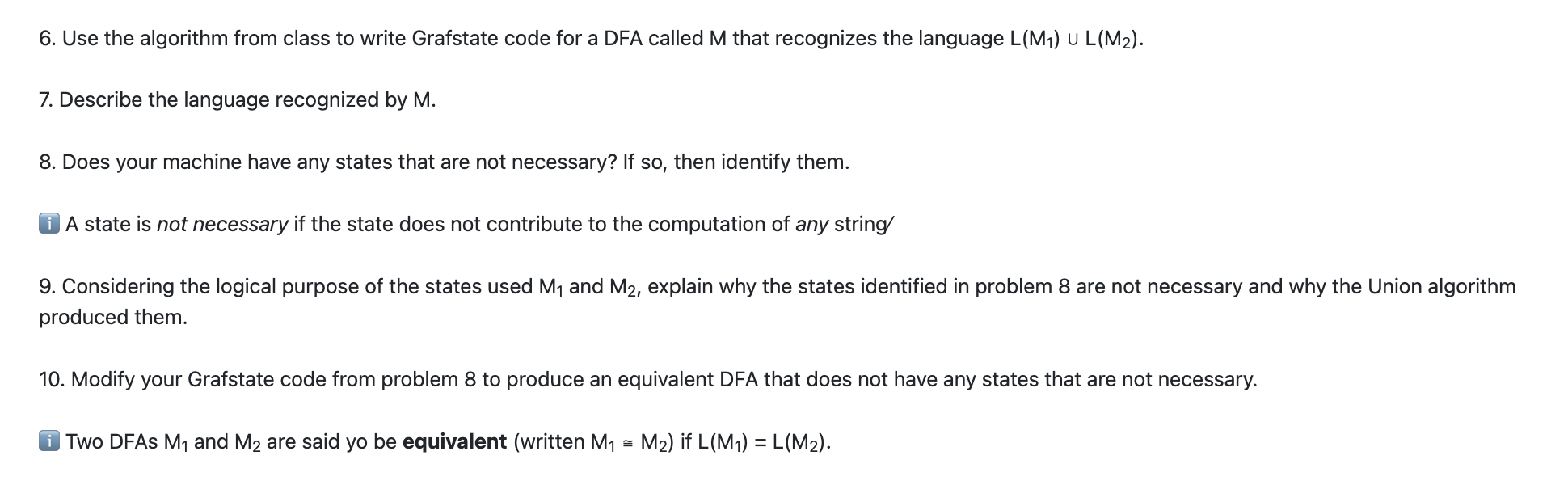Home / Expert Answers / Computer Science / 6-use-the-algorithm-from-class-to-write-grafstate-code-for-a-dfa-called-m-that-recognizes-t-pa123

# (Solved): 6. Use the algorithm from class to write Grafstate code for a DFA called $$M$$ that recognizes t ...6. Use the algorithm from class to write Grafstate code for a DFA called $$M$$ that recognizes the language $$L\left(M_{1}\right) \cup L\left(M_{2}\right)$$. 7. Describe the language recognized by M. 8. Does your machine have any states that are not necessary? If so, then identify them. A state is not necessary if the state does not contribute to the computation of any string 9. Considering the logical purpose of the states used $$M_{1}$$ and $$M_{2}$$, explain why the states identified in problem 8 are not necessary and why the Union algorithm produced them. 10. Modify your Grafstate code from problem 8 to produce an equivalent DFA that does not have any states that are not necessary. Two DFAs $$M_{1}$$ and $$M_{2}$$ are said yo be equivalent (written $$\left.M_{1} \cong M_{2}\right)$$ if $$L\left(M_{1}\right)=L\left(M_{2}\right)$$.

We have an Answer from Expert